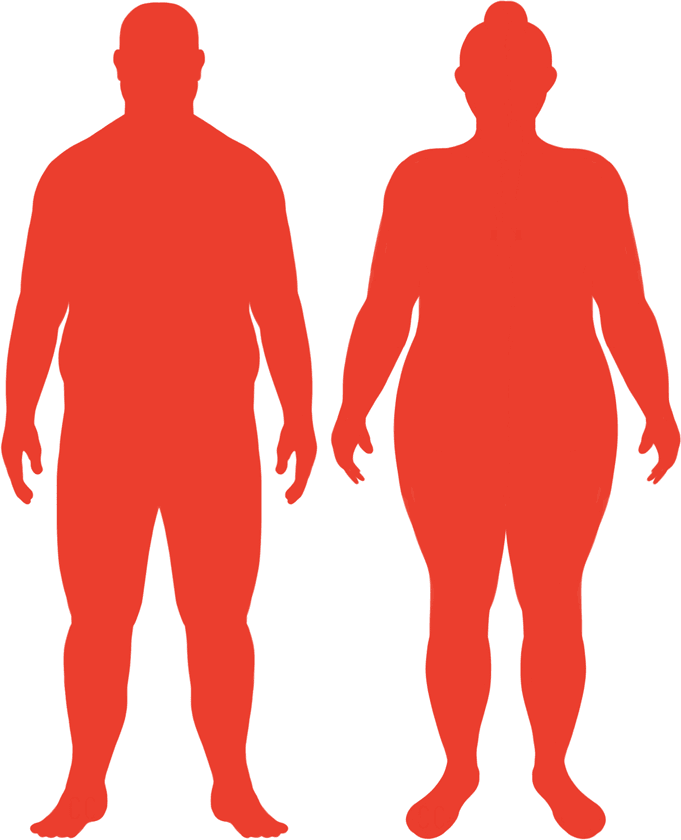# BMI Calculator

Result for 7'7" and 428 lbs.
Change Here.36.33

### Obese BMI

At 7'7" and 428 Pounds your BMI is 36.33

### BMI Scale

 Under Normal Over Obese
-75 lbs
to reach an
overweight bmi classification.
##### Overweight BMI Ends:
353.4 lbs
-134 lbs
to reach a
normal bmi classification.
294.5 lbs

### BMI Grading Table2 for Height 7'7"

 Weight Weight Class Below 217.9 lbs Underweight 217.9 lbs - 294.5 lbs Normal Weight 294.5 lbs - 353.4 lbs Overweight 353.4 lbs and Above Obese

### Weight Loss

If you were seeking a normal BMI weight of 294.5lbs, and you lost two pounds a week4, then you could reach a normal BMI in
67 Weeks
Or to be considered overweight, a goal weight of 353.4 lbs could be reached in
38 Weeks

### Obese BMI Silhouette### Ideal Weight for a 7 foot 7 Male or Female2

The ideal weight range using the BMI for a male or female with a height of 7'7" is

### Between

217.9 lbs

and
294.5 lbs
How to Calculate BMI3 for 7'7" and 428 lbs
(7 x 12) + 7 = 91
(428 / (91 x 91)) x 703
= 36.334259147446
7 = foot | 7 = inch | 428 = weight lbs

### Conversion

• 7 foot 7 = 91 inches
• 7 foot 7 = 231.14cm
• 428 pounds = 30 stone 8 pounds
• 428 pounds = 194.14 kg
• 428 pounds = 194137.53 grams

### 7'7" and 428 lbs Summary

What is the BMI for a 7'7" and 428 lbs female? 36.33 BMI, Obese.
What is the BMI for a 7'7" and 428 lbs male? 36.33 BMI, Obese.
What is the ideal weight for a 7'7" female? Between: 217.9lbs and 294.5lbs
What is the ideal weight for a 7'7" male? Between: 217.9lbs and 294.5lbs
If I am 7ft 7in and weigh 428 lbs, is that a good weight for my height? Under the BMI classification, 428 lbs is classed as being Obese.